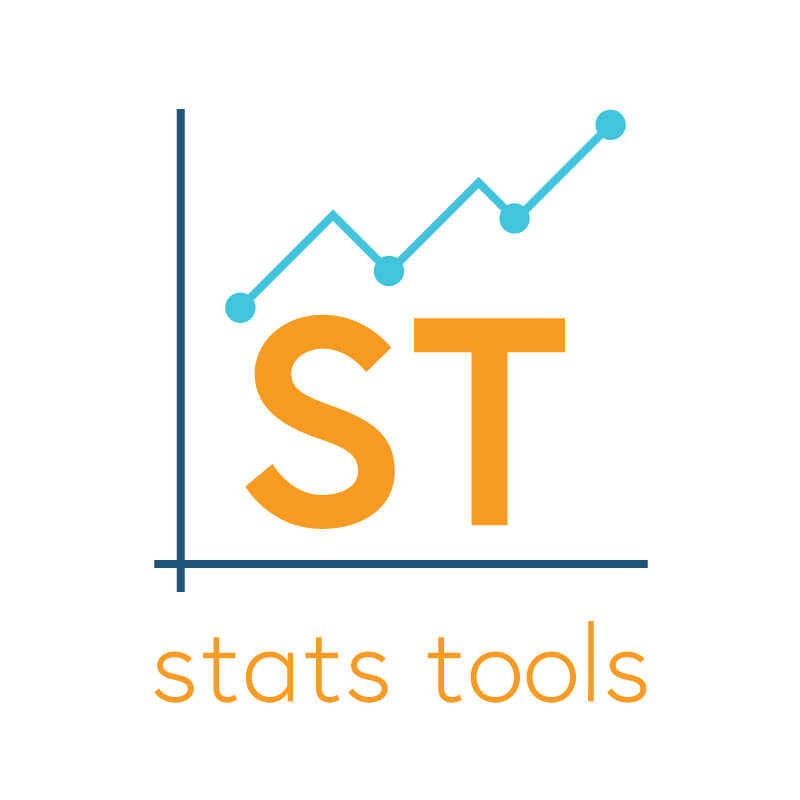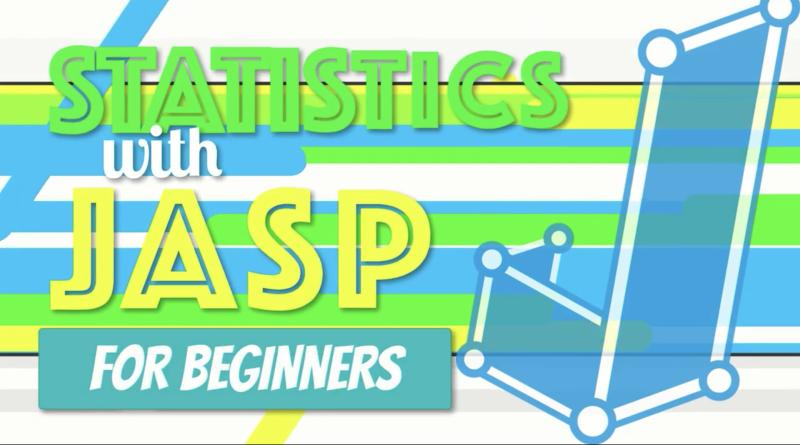# 15. Regression## Resources: Stats of Doom

A comprehensive site created by Dr. Erin Buchanan offering lecture materials and videos for a full range of statistics concepts covered in a basic/introductory course (descriptives, graphs, z-scores, t-tests, ANOVA, correlation, regression, chi-square, etc.). Coverage also includes materials for several statistics packages (e.g., SPSS, JASP, R) Here is a link for a basic course. There …## Video: How to Do Simple Linear Regression in JAS

Video (23:18) from Research by Design in which, “Exploring our data about burnout and job satisfaction, we predict an outcome with a single variable using simple linear regression in JASP. I explain how regression works, then open an SPSS dataset in JASP. We explore the assumptions of homoscedasticity and linearity with a scatterplot and normality …## Tutorial: Regression (WISE)

Online tutorial that guides students through a research study and data analysis using regression. There are 4 modules total, with the first three being most appropriate for an introductory course. Module 3 is short and could be used as an in-class demonstration. Module 4 is more advanced. During the tutorial students will start with a …

## Design & Data: Is Going Greek a Good Idea? (Survey Research and Correlation)

Key Topics and Links to Files Data Analysis Guide Is Going Greek a Good Idea? Analyses Included: Pearson r correlation; Descriptive Statistics (Frequencies; Central Tendency); Scale Reliability (Cronbach’s alpha); Creating a Sum Dataset Syntax Output BONUS: What Contributes to College Satisfaction? Analyses Included: Pearson r correlation; Multiple Regression Dataset Syntax Output Design Summary (for the …

## Open Stats Lab: Regression Activities

Activities focus on the following topics: Why are People Biased When Reasoning About the Status Quo? Here students get to conduct bivariate correlations, regression, and multiple regression. They may also create a table. Do Experts Overrate the Extent of Their Expertise? Here students will conduct descriptive statistics, t-tests, multiple regression, and use a split file. …## Lecture Slides: Predicting Job Performance (Multiple Regression)

This is an example (created by Dr. Alisa McArthur) looking at predictors of job performance that shows simple multiple regression using SPSS which illustrates how multicollinearity can lead to results that are inconsistent and difficult to interpret. It includes both a PowerPoint as well as hard copy for students to help them get more comfortable …